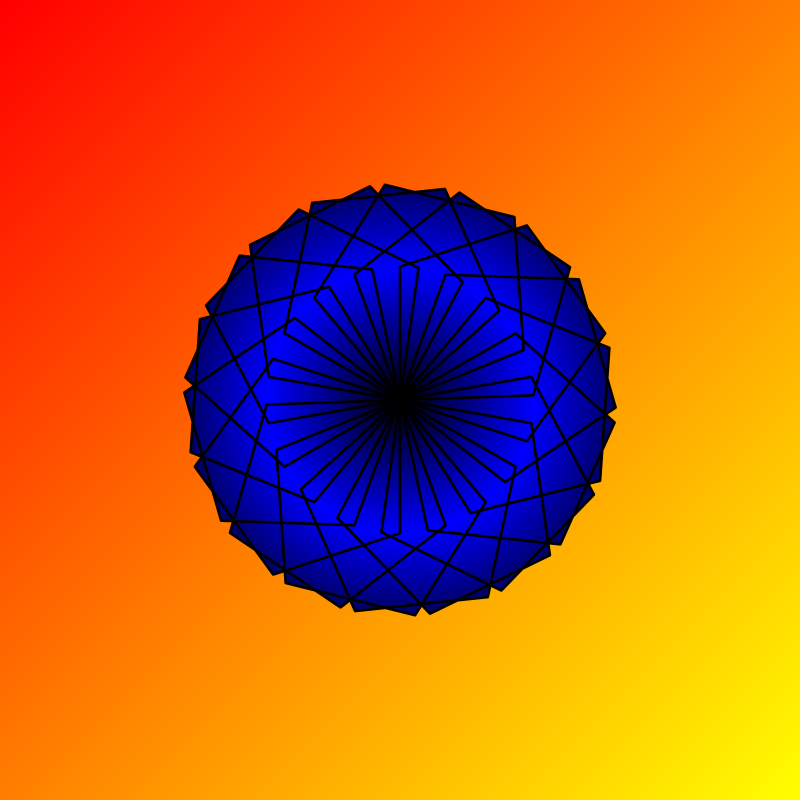## docs for Kojo

This activity has the following desired goals:

• Learning to apply the above ideas to make pleasing drawings (M, T).

### Step 1

Type in the following code and run it:

``````clear()
setSpeed(fast)
val cb = canvasBounds
val clr = cm.linearGradient(cb.x, cb.y, red, cb.x + cb.width, cb.y + cb.height, yellow, true)
setBackground(clr)
``````

Q1a. What do you think is a linear color gradient?

Q1b. The above code creates a gradient from which point in the canvas to which point?

Q1c. What is the start color of the gradient? What is the end color?

### Explanation

Function description:

• `cm.linearGradient(x1, y1, c1, x2, y2, c2, cyclic)` - Creates a linear color gradient between color `c1` at position `(x1, y1)` and color `c2` at position `(x2, y2)`. The `cyclic` parameter specifies whether the gradient should be repeated or not.

### Exploration

Create a linear gradient to fill a rectangle.

### Step 2

Type in the following code and run it:

``````clear()
setSpeed(fast)
val cb = canvasBounds
setBackground(clr)
``````

Q2b. The above code creates a gradient from which point in the canvas to which points?

Q2c. What is the start color of the gradient? What is the end color?

### Explanation

Function description:

• `cm.radialGradient(cx, cy, c1, radius, c2, cyclic)` - Creates a radial color gradient between color `c1` at the center of a circle positioned at `(x1, y1)` and color `c2` at a distance `radius` from the center. The `cyclic` parameter specifies whether the gradient should be repeated or not.

### Exploration